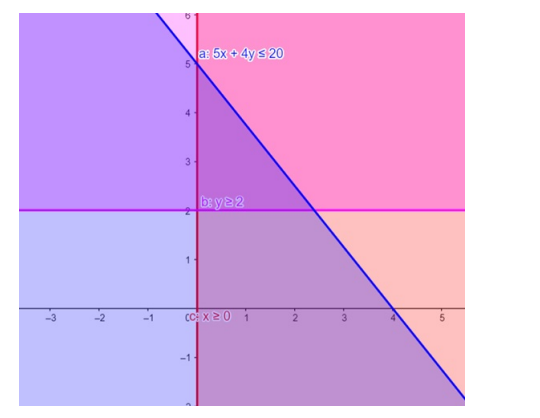# Solve the given inequalities

Question:

Solve the given inequalities $5 x+4 y \leq 20, x \geq 1, y \geq 2$ graphically in two - dimensional plane.

Solution:

The graphical representation of $5 x+4 y \leq 20, x \geq 1, y \geq 2$ is given by common region in the figure below.

$5 x+4 y \leq 20$ .…… (1)

$x \geq 1 \ldots \ldots .(2)$

$y \geq 2 \ldots \ldots .(3)$

Inequality $(1)$ represents the region below line $5 x+4 y=20$ (including the line $5 x+4 y=20$ ).

Inequality (2) represents the region in front of line $x=1$ (including the line $x=1$ ).

Inequality (3) represents the region above line $y=2$ (including the line $y=2$ ).

Therefore,every point in the common shaded region including the points on the respective lines represents the solution for the given inequalities.

This can be represented as follows,